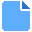# Mathwizard2.5

Developed by - Windows - setup file Mathwizardsetup25.zip (0.59 MB) - Shareware

MD5: 9c5799125fe29d948e01f6c6d14ff260

## Developer Description

"most powerful calculator"

It is one of the most powerful math tools there is. It gathers between the simplicity and ease of use of a simple calculator and the ability to solve complex math procedures.
Here is a brief description of the exciting capabilities of mathwizard:
1. Matrices :

>For real and complex matrices.
>for real matrices matrix dimensions can be up to 12x12 and for complex matrices matrix dimensions can be up to 10x10 .
>Can store three matrices internally
>Programmable
>shows the past history of manipulations on matrices with their results
>Can do all manipulations on real and complex matrices. It can do add, subtract, multiply, transpose, multiply or divide by a constant, inverse, determinant, and solve a system of simultaneous equations,LU factorization and can find rank.
>Very easy exciting and fast to use

2. Algebra :
> >Can store up to three algeriac equations internally
>Programmable
>It can do the operations of add, subtract, multiply, and divide of any two algebraic equations algebraically and produce an algebraic result, it can also multiply and divide an algebraic function with constant, it is a smart peace of software and can factorize any algebraic function as well as find the roots, finally it can evaluate an algebraic expression.
>Very easy exciting and fast to use

3. Plot graph :
>Can plot up to three graphs simultaneously.
>You enter the mathematics expressions in the same way you write it at school and university.It supports brackkets and nested brackets as well as trigonometric, exponential , and logarithmic functions
4. Integration :
>Numeric evaluation of integration
>One line text editor that supports mathematic expressions.
5. Differentiation :
>Numeric evaluation of Diferentation
>One line text editor that supports mathematic expressions.
6. Scientific calculator :
>Numeric evaluation of a function
>One line text editor that supports mathematic expressions.

Requirements: DotVetFrameWork 4.0

What's new in this version: better user experience

### Antivirus information

Download3K has downloaded and tested Mathwizard on 5 Feb 2014 with some of Today's best antivirus engines and you can find below, for your convenience, the scan results:

Clean

Clean

Clean

Clean
User rating